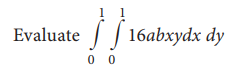Home | | Statistics 11th std | Double integrals

# Double integrals

A double integral is an integral of two variable function f(x,y) over a region R If R=[a, b] × [c, d] then the double integral can be done by iterated Integration.

Double integrals

A double integral is an integral of two variable function f(x,y) over a region R If R=[a, b] × [c, d] then the double integral can be done by iterated Integration( integrate first with respect to y and then with respect to x )

The notation used for double integral isHere the function f(x,y) is integrated with respect to y first and treat f(x) constant and then integrate with respect to x and apply limits of x and simplify

### Example 7.23### Solutions:

Let us first integrate with respect to y and then with respect to x. Hence the double integral is written as### Example 7.24### Solution:### Example 7.25### Solution:Tags : Formula, Solved Example Problems , 11th Statistics : Chapter 7 : Mathematical Methods
Study Material, Lecturing Notes, Assignment, Reference, Wiki description explanation, brief detail
11th Statistics : Chapter 7 : Mathematical Methods : Double integrals | Formula, Solved Example Problems